# BAIIISemEconomics Prepared ByDr Suneyna Department of Economics Meaning

• Slides: 19
Download presentation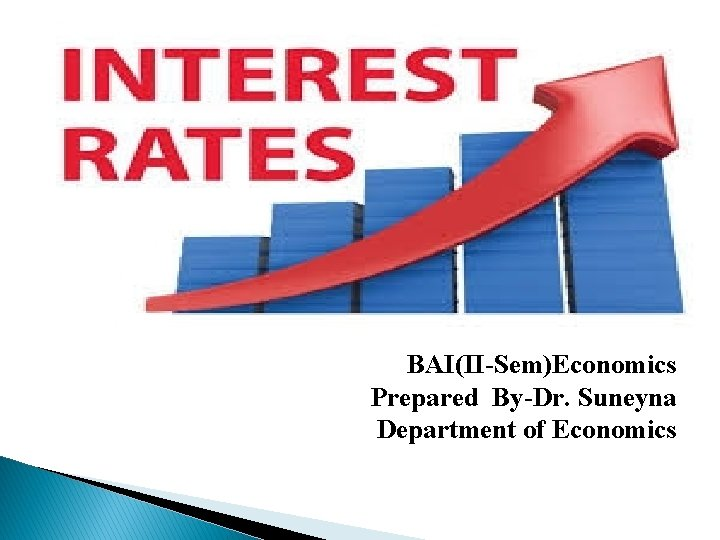BAI(II-Sem)Economics Prepared By-Dr. Suneyna Department of Economics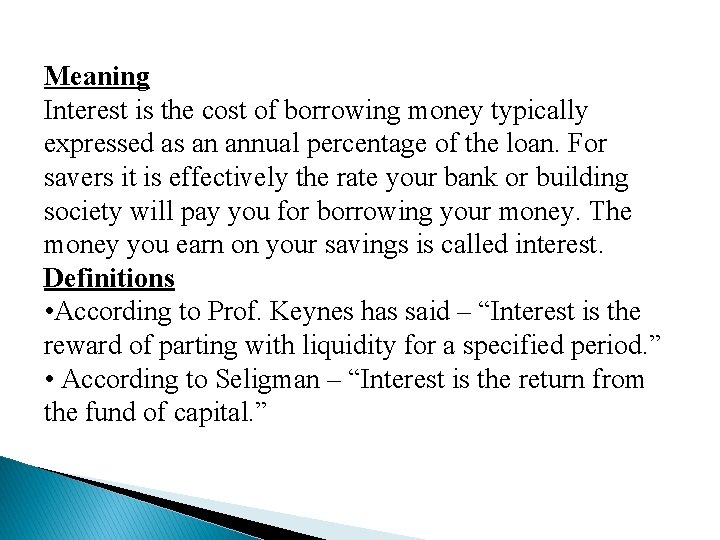Meaning Interest is the cost of borrowing money typically expressed as an annual percentage of the loan. For savers it is effectively the rate your bank or building society will pay you for borrowing your money. The money you earn on your savings is called interest. Definitions • According to Prof. Keynes has said – “Interest is the reward of parting with liquidity for a specified period. ” • According to Seligman – “Interest is the return from the fund of capital. ”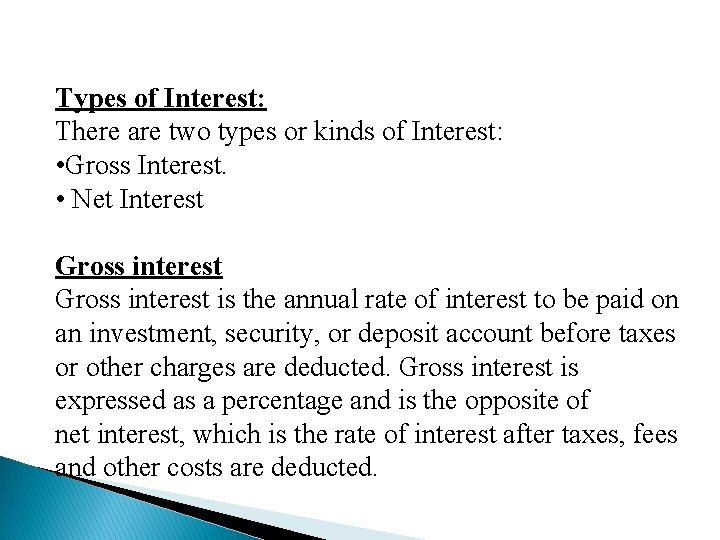Types of Interest: There are two types or kinds of Interest: • Gross Interest. • Net Interest Gross interest is the annual rate of interest to be paid on an investment, security, or deposit account before taxes or other charges are deducted. Gross interest is expressed as a percentage and is the opposite of net interest, which is the rate of interest after taxes, fees and other costs are deducted.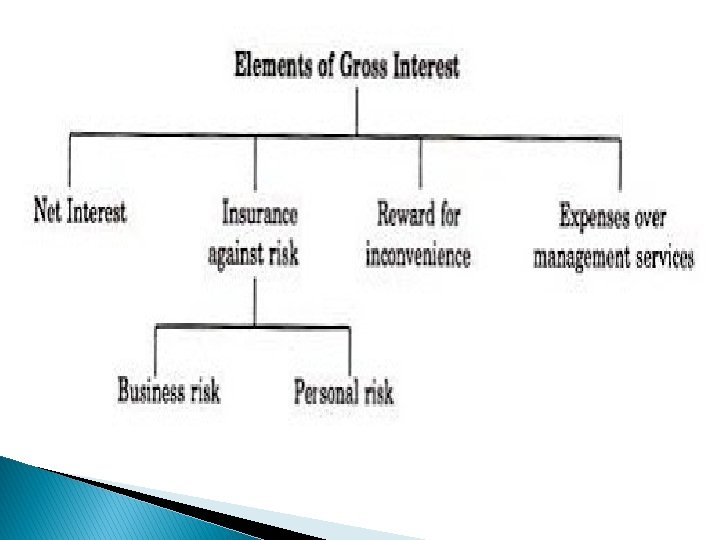Thus Gross Interest = Net Interest + Payment for risk + Payment for management services + Compensation for the changing value of money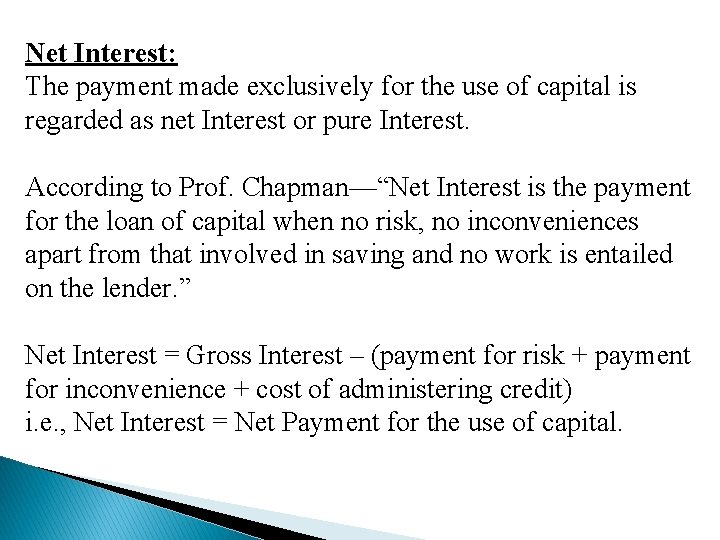Net Interest: The payment made exclusively for the use of capital is regarded as net Interest or pure Interest. According to Prof. Chapman—“Net Interest is the payment for the loan of capital when no risk, no inconveniences apart from that involved in saving and no work is entailed on the lender. ” Net Interest = Gross Interest – (payment for risk + payment for inconvenience + cost of administering credit) i. e. , Net Interest = Net Payment for the use of capital.The Classical Theory of Interest The classical theory of interest also known as the demand supply theory was propounded by the economists like Marshall and Fisher. Later on, Pigou, Cassel, Knight and Taussig worked to modify theory. The Classical theory is also called 'Real' theory of interest, because it is based on real forces of demand supply side, i. e. , productivity on the side of demand thrift on the side of supply. Determination of Interest= Investment (Demand Side) =Saving (Supply Side)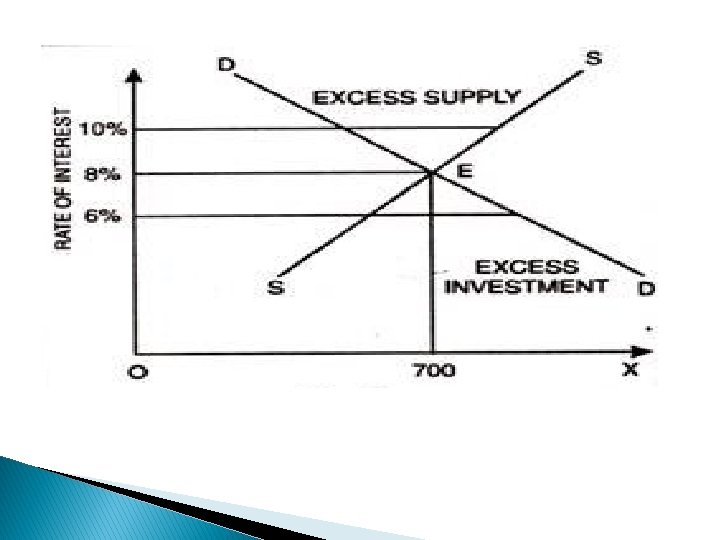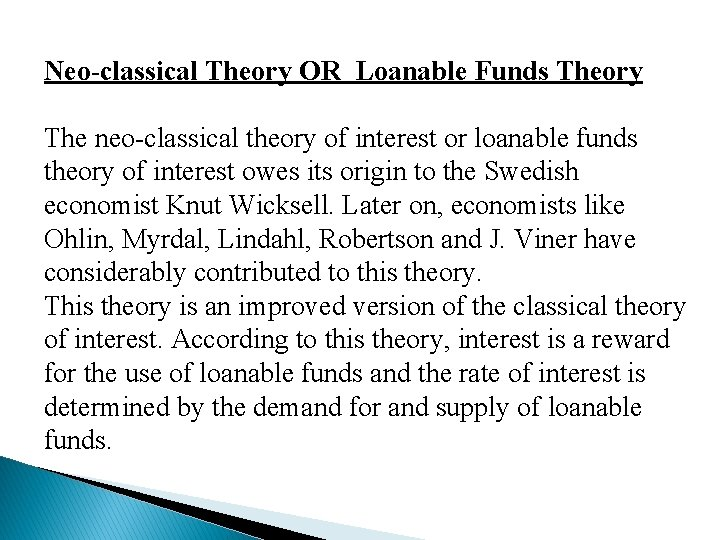Neo-classical Theory OR Loanable Funds Theory The neo-classical theory of interest or loanable funds theory of interest owes its origin to the Swedish economist Knut Wicksell. Later on, economists like Ohlin, Myrdal, Lindahl, Robertson and J. Viner have considerably contributed to this theory. This theory is an improved version of the classical theory of interest. According to this theory, interest is a reward for the use of loanable funds and the rate of interest is determined by the demand for and supply of loanable funds.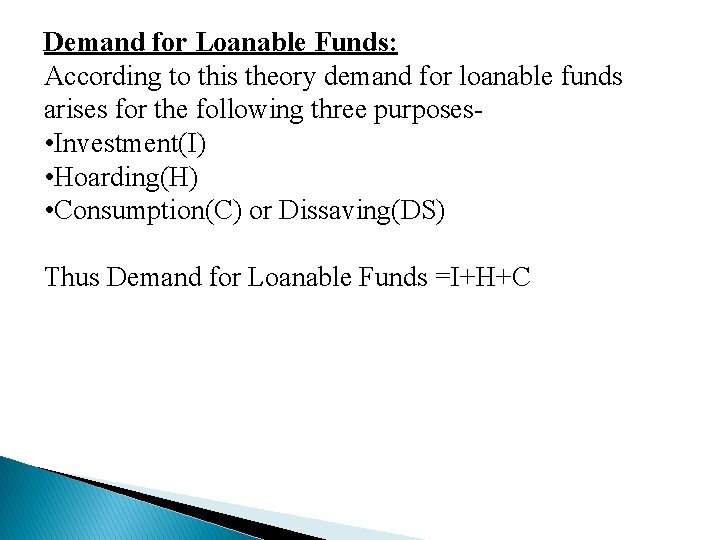Demand for Loanable Funds: According to this theory demand for loanable funds arises for the following three purposes • Investment(I) • Hoarding(H) • Consumption(C) or Dissaving(DS) Thus Demand for Loanable Funds =I+H+CSupply of Loanable Funds: The supply of loanable funds is derived from the following basic four sources- • Savings(S) • Dishoarding(DH) • Disinvestment (DI) • Bank Credit(B) or Bank. Money(BM) Thus Supply of Loanable Funds =S+DH+DI+B Determination of Interest= Demand for Loanable Funds + Supply of Loanable Funds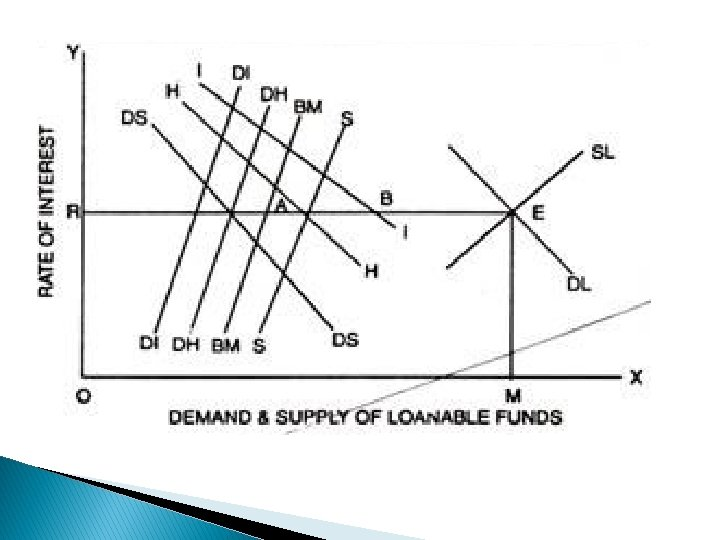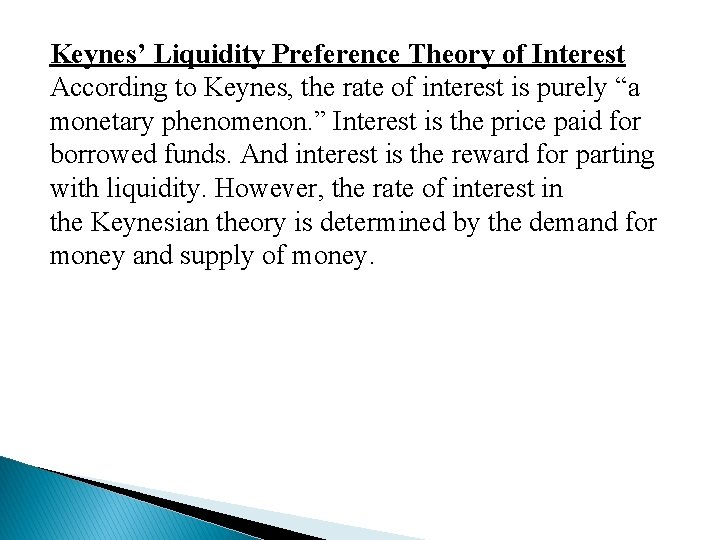Keynes’ Liquidity Preference Theory of Interest According to Keynes, the rate of interest is purely “a monetary phenomenon. ” Interest is the price paid for borrowed funds. And interest is the reward for parting with liquidity. However, the rate of interest in the Keynesian theory is determined by the demand for money and supply of money.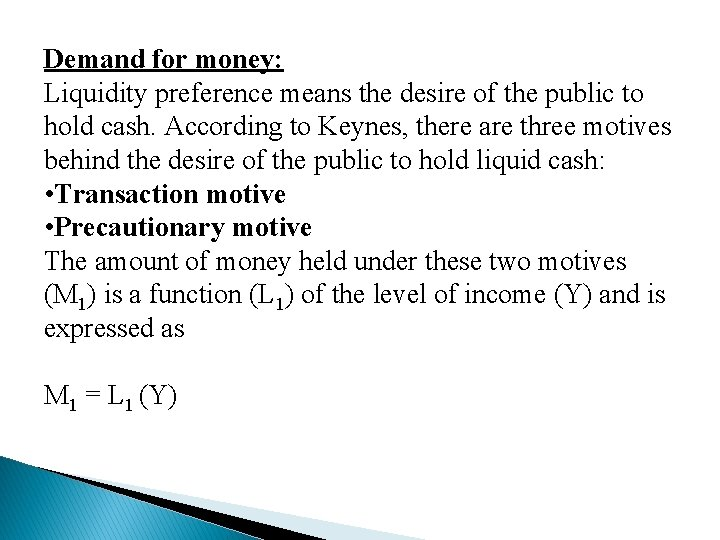Demand for money: Liquidity preference means the desire of the public to hold cash. According to Keynes, there are three motives behind the desire of the public to hold liquid cash: • Transaction motive • Precautionary motive The amount of money held under these two motives (M 1) is a function (L 1) of the level of income (Y) and is expressed as M 1 = L 1 (Y)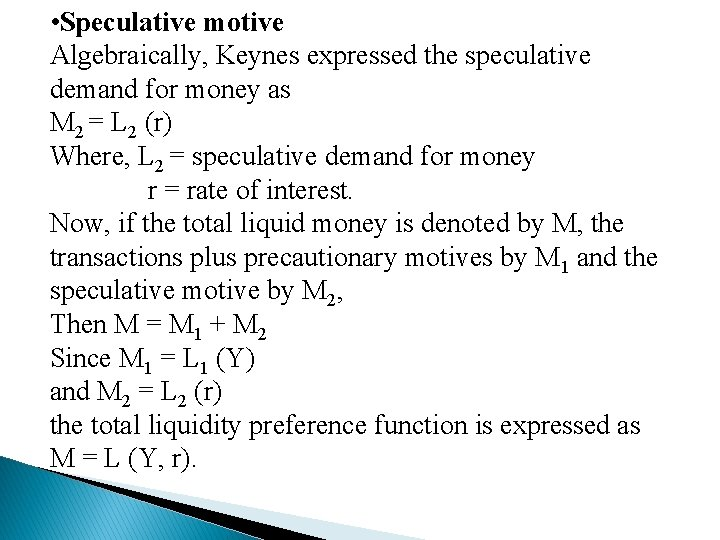• Speculative motive Algebraically, Keynes expressed the speculative demand for money as M 2 = L 2 (r) Where, L 2 = speculative demand for money r = rate of interest. Now, if the total liquid money is denoted by M, the transactions plus precautionary motives by M 1 and the speculative motive by M 2, Then M = M 1 + M 2 Since M 1 = L 1 (Y) and M 2 = L 2 (r) the total liquidity preference function is expressed as M = L (Y, r).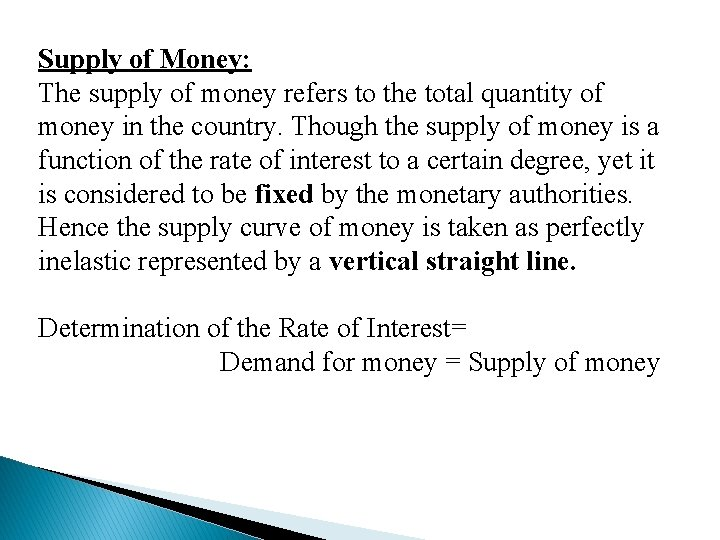Supply of Money: The supply of money refers to the total quantity of money in the country. Though the supply of money is a function of the rate of interest to a certain degree, yet it is considered to be fixed by the monetary authorities. Hence the supply curve of money is taken as perfectly inelastic represented by a vertical straight line. Determination of the Rate of Interest= Demand for money = Supply of money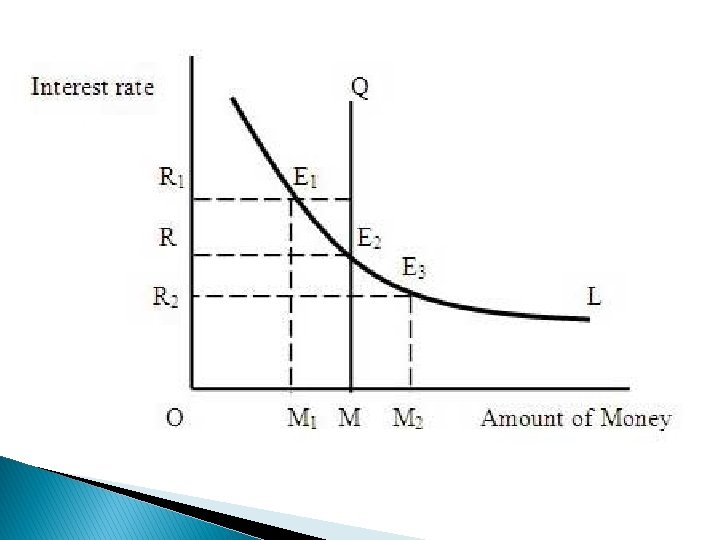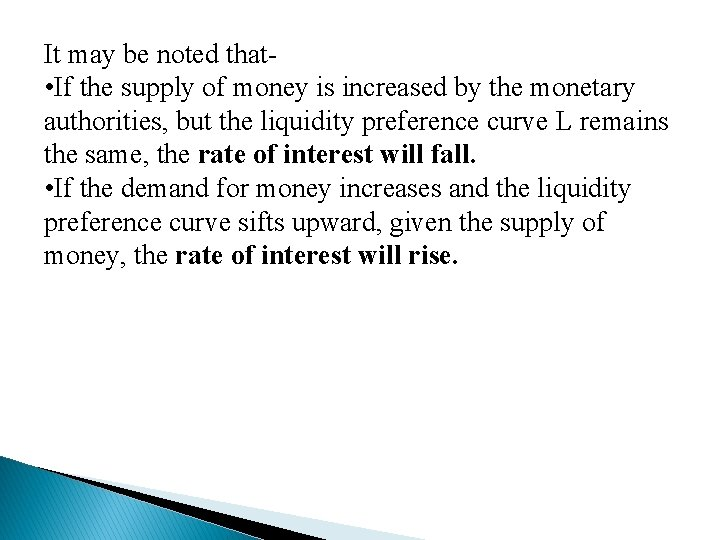It may be noted that • If the supply of money is increased by the monetary authorities, but the liquidity preference curve L remains the same, the rate of interest will fall. • If the demand for money increases and the liquidity preference curve sifts upward, given the supply of money, the rate of interest will rise.THANK YOU